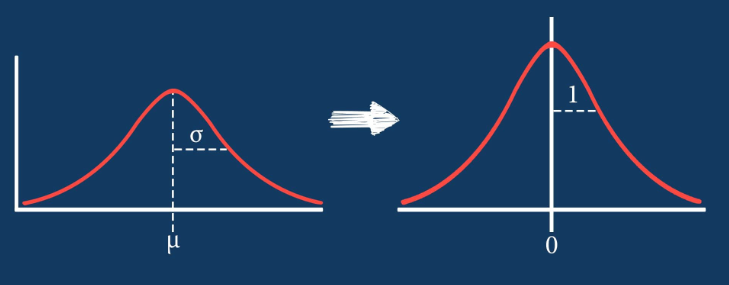Join thousands of AI enthusiasts and experts at the Learn AI Community.

## Publication# Machine Learning Standardization (Z-Score Normalization) with Mathematics

Last Updated on November 17, 2021 by Editorial Team

### Introduction

In Machine Learning, feature scaling is very important and a dime a dozen because it makes sure that the features of the data-set are measured on the same scale. The concept of feature scaling has come to the fore from statistics. It is an approach to plonk different variables on the same scale. It is commonly used when data-set has varying scales. Sometimes the features of data-set use to have exhaustive and large differences between their ranges. So, in this case, standardization overawes on the data-set to bring all on the same scale.

This concept is extensively used in SVM, Logistic Regression, and Neural Network.

The concept of Standardization (Z-Score Normalization) is completely based on the mathematical concepts called Standard Derivation and Variance.

### Variance

The variance is the average of the squared difference from the mean.

Below are steps to derive variance:

• Calculate the mean
• Subtract the value of mean from each number
• Square the subtracted result

Equation:

Example:

Let’s there are different categories of cats with their height (mm).

Here, A = Type of cat and B = Height of cat

Calculate the variance of cats’ height:

Now, calculate each cat’s height difference from the derived mean:

Calculate variance:

### Standard Deviation

Standard Deviation is the square root of variance.

Equation of Standard Deviation:

Now, calculate standard deviation from the value of variance:

This derived value says that which cat’s height is within this standard deviation range.

### Standardization (Z-Score Normalization)

Standardization is the concept and step of putting different variables on the same scale. This concept allows comparing scores between different types of variables.

Equation of Standardization:

### An Example where Standardization is used

Lets there are two-dimensional data-set with two different features — height and BMI.

Height is in inch and BMI is in its value and here the value of height is very large in comparison with BMI. So, height will dominate over the BMI feature and will have more contribution to the distance computation.

Height(inch) = [165, 172]

BMI = [18.5. 25]

This problem can be solved by applying the technique of Standardization (Z-Score Normalization).

### Conclusion

There are two common approaches to bringing different features onto the same scale: normalization and standardization. Most often, normalization refers to the rescaling of the features to a range of [0, 1], which is a special case of min-max scaling. Using standardization, we center the feature columns at mean 0 with standard deviation 1 so that the feature columns take the form of a normal distribution, which makes it easier to learn the weights. Standardization maintains useful information about outliers and makes the algorithm less sensitive to them in contrast to min-max scaling.Machine Learning Standardization (Z-Score Normalization) with Mathematics was originally published in Towards AI — Multidisciplinary Science Journal on Medium, where people are continuing the conversation by highlighting and responding to this story.

Published via Towards AI